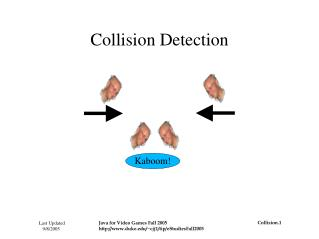DownloadDownload PresentationCollision Detection

# Collision Detection

Download Presentation## Collision Detection

- - - - - - - - - - - - - - - - - - - - - - - - - - - E N D - - - - - - - - - - - - - - - - - - - - - - - - - - -
##### Presentation Transcript

1. Collision Detection Kaboom!

2. The Plan • What is collision detection? • Why is collision detection important? • Classification of collision detection • Tools for finding collisions • How and where collision detection is implemented in the video game package

3. What is collision detection? Collision detection is determining the time and/or location of the intersection of two or more objects. Kaboom!

4. Why is collision detection important? • Simulations • air traffic control • physics • video games • Modeling • molecular models • packing algorithms • Computer Aided Design (CAD)

5. Classification of Collision Detection • Type of objects • convexity/concavity • rigid/deformable • polygons/curves • 1-Dimensional/2D/3D/N-D • static/dynamic • Time of collision • discrete/continuous • static/dynamic • exact/approximate • Motion of objects • linear/non-linear • predictable/dynamic • bounded/unbounded • angular motion • Other factors • realtime/off-line • number of objects • allowable error margin • frame vs. path

6. Tools for finding collisions • Bounding boxes/circles/spheres • Constructive Area Geometry • Spacial separation trees • Tile maps • Numerical analysis methods

7. Collision Detection in theVideo Game Package • Default uses a single bounding box • Images must use a bounding box • CAG used for approximations • All objects are polygons (or approximated by polygons) • No pruning other than use of bounding box for first approximation

8. Collision Detection in theVideo Game Package For pair (j, k) of Sprites: • if the boundingBox(j) does not intersect boundingBox(k), return no intersection • let m be the intersection of j and k • if m is empty, return no intersection • otherwise, return intersection • This algorithm uses a simple test for intersection when possible (intersection of bounding boxes), and a more exact test when the bounding box fails to determine intersection.

9. Collision Detection in theVideo Game Package What this collision detection is not good at doing: • determining path intersections (important for fast moving objects or low model frame rates) • determining time/location of first intersection • predicting intersections • handling a large number of Sprites

10. Collision Detection in theVideo Game Package Location & Response of collision detection • Sprite • public void setUseBoundingBox(boolean box) • public boolean intersects(Sprite sprite) • public static double getNormalVector(Shape stationary, Shape moving) • ProjectileTracker • public void bounce(double normal)